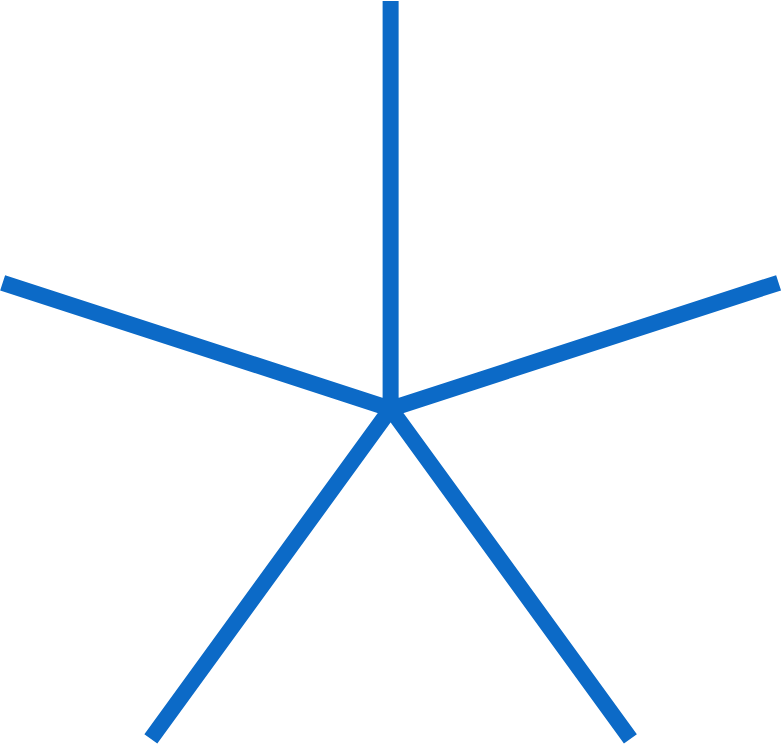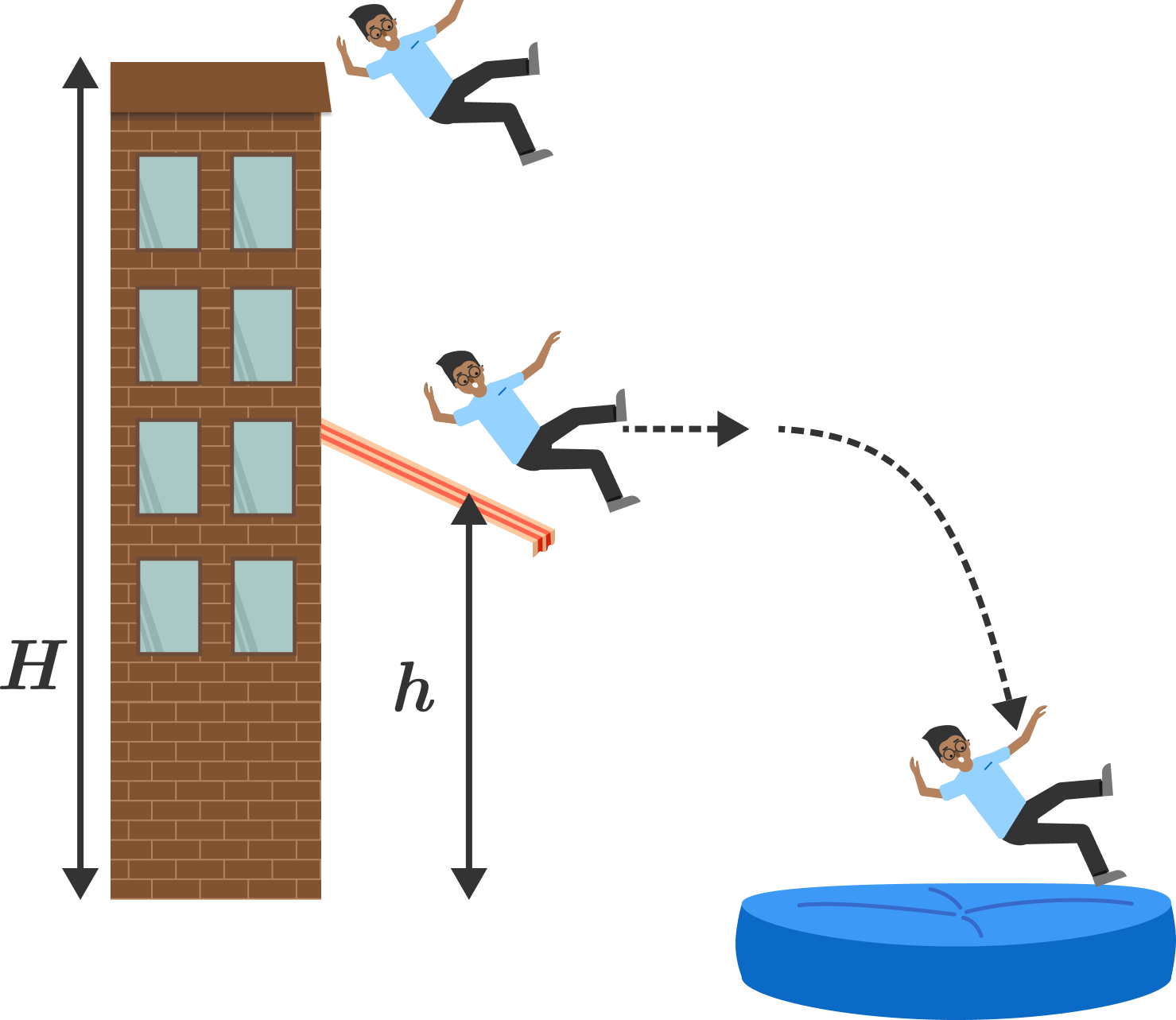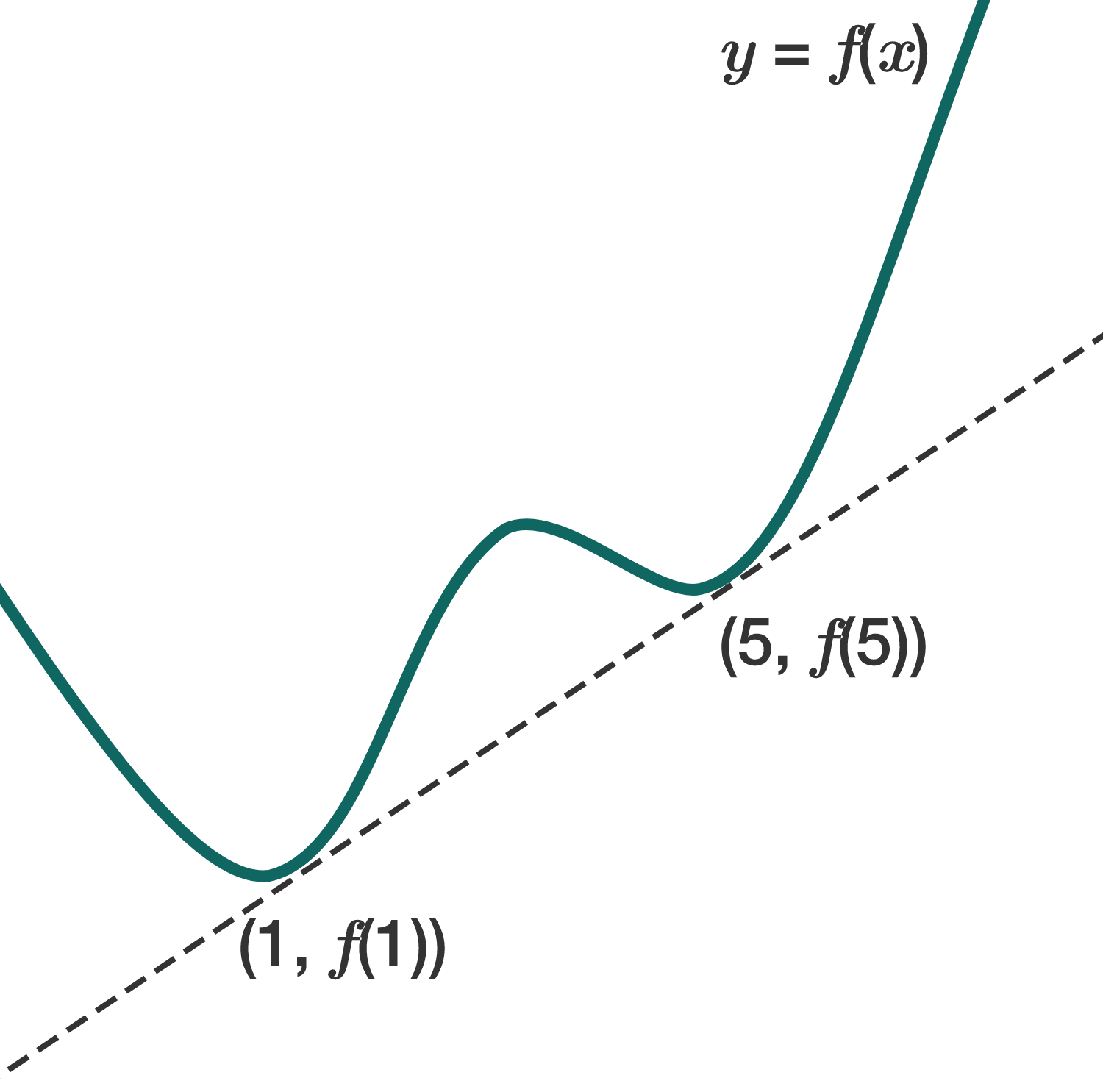# Problems of the Week

Contribute a problem

# 2018-11-05 IntermediateThis star has five spokes that are each $1$ unit long, and the angles between adjacent spokes are all the same.

How many copies of this star would you need to completely cover a bigger star with spokes of length $1.1?$

Inspiration

Pierre in Paris $(48.9 ^\circ \text{ N}, 2.3 ^\circ \text{ E})$ and Sadie in Sydney $(33.9 ^\circ \text{ S}, 151.2 ^\circ \text{ E})$ each perform the following experiment:

• Set a pendulum swinging exactly along the East-West direction.
• As Earth rotates about its axis, the plane of oscillation of the pendulum slowly rotates. Eventually, the pendulum will be momentarily swinging along the North-South direction.
• Measure the amount of time it takes for this to happen for the first time.

Whose pendulum will take a greater amount of time?A stuntman falls from the top of a building of height $H$. On his way down, he bounces off of an awning at a height of $h$ in a perfectly elastic collision. Immediately after the bounce, the stuntman moves horizontally.

For what value of $\frac hH$ will the man hit the safety cushion (at ground level) the farthest from the building?

Assume that air resistance is negligible.

Inspiration

\begin{aligned} 144 &= 12^2 \\ 1444 &= 38^2 \end{aligned}

Are there any other numbers $144 \ldots 44$ (starting with 1 and followed by all 4s) that are perfect squares?

The function $f(x)$ is defined for real constants $a,$ $b,$ $c,$ and $d:$

$f(x)=x^4+ax^3+bx^2+cx+d.$

The graph of the function is shown below.

Given that the tangent lines at $\big(1,f(1)\big)$ and at $\big(5,f(5)\big)$ coincide, what is the value of $a+b?$×